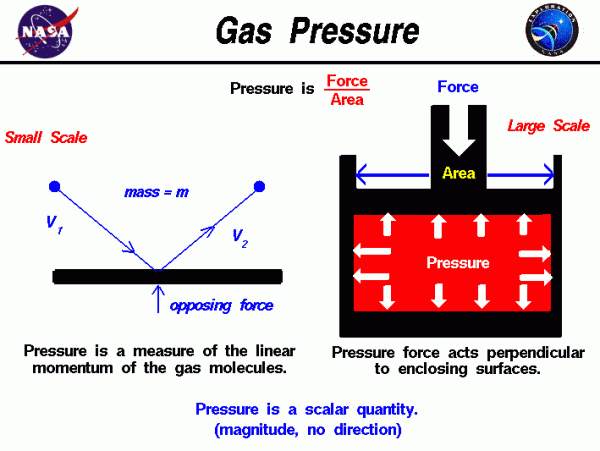# Gas Pressure## Pressure – An important essential

An important property of any gas is its pressure. We have some experience with gas pressure that we don’t have with properties like viscosity and compressibility. Every day we hear the TV meteorologist give value of the barometric pressure of the atmosphere (29.8 inches of mercury, for example). And most of us have blown up a balloon or used a pump to inflate a bicycle tire or a basketball.

Because understanding what pressure is and how it works is so fundamental to the understanding of rocketry, we are including several slides on air pressure in the Beginner’s Guide. An interactive atmosphere simulator allows you to study how static air pressure changes with altitude. The RocketModeler II program shows you how atmospheric pressure effects the flight of rockets, and the Rocket Thrust program shows how the pressure changes through a rocket nozzle. Another simulator helps you study how pressure changes across shock waves that occur at high speeds. There are two ways to look at pressure: (1) the small scale action of individual air molecules or (2) the large scale action of a large number of molecules.

## Molecular Definition of Pressure

From the kinetic theory of gases, a gas is composed of a large number of molecules that are very small relative to the distance between molecules. The molecules of a gas are in constant, random motion and frequently collide with each other and with the walls of any container. The molecules possess the physical properties of mass, momentum, and energy. The momentum of a single molecule is the product of its mass and velocity, while the kinetic energy is one half the mass times the square of the velocity. As the gas molecules collide with the walls of a container, as shown on the left of the figure, the molecules impart momentum to the walls, producing a force perpendicular to the wall. The sum of the forces of all the molecules striking the wall divided by the area of the wall is defined to be the pressure. The pressure of a gas is then a measure of the average linear momentum of the moving molecules of a gas. The pressure acts perpendicular (normal) to the wall; the tangential (shear) component of the force is related to the viscosity of the gas.

## Scalar Quantity

Let us look at a static gas; one that does not appear to move or flow. While the gas as a whole does not appear to move, the individual molecules of the gas, which we cannot see, are in constant random motion. Because we are dealing with a nearly infinite number of molecules and because the motion of the individual molecules is random in every direction, we do not detect any motion. If we enclose the gas within a container, we detect a pressure in the gas from the molecules colliding with the walls of our container. We can put the walls of our container anywhere inside the gas, and the force per area (the pressure) is the same. We can shrink the size of our “container” down to an infinitely small point, and the pressure has a single value at that point. Therefore, pressure is a scalar quantity, not a vector quantity. It has a magnitude but no direction associated with it. Pressure acts in all directions at a point inside a gas. At the surface of a gas, the pressure force acts perpendicular to the surface.

## Macro Scale Definition of Pressure

Turning to the larger scale, the pressure is a state variable of a gas, like the temperature and the density. The change in pressure during any process is governed by the laws of thermodynamics. You can explore the effects of pressure on other gas variables at the animated gas lab. Although pressure itself is a scalar, we can define a pressure force to be equal to the pressure (force/area) times the surface area in a direction perpendicular to the surface. The pressure force is a vector quantity.

Pressure forces have some unique qualities as compared to gravitational or mechanical forces. In the figure shown above on the right, we have a red gas that is confined in a box. A mechanical force is applied to the top of the box. The pressure force within the box opposes the applied force according to Newton’s third law of motion. The scalar pressure equals the external force divided by the area of the top of the box. Inside the gas, the pressure acts in all directions. So the pressure pushes on the bottom of the box and on the sides. This is different from simple solid mechanics. If the red gas were a solid, there would be no forces applied to the sides of the box; the applied force would be simply transmitted to the bottom. But in a gas, because the molecules are free to move about and collide with one another, a force applied in the vertical direction causes forces in the horizontal direction.

Provide feedback